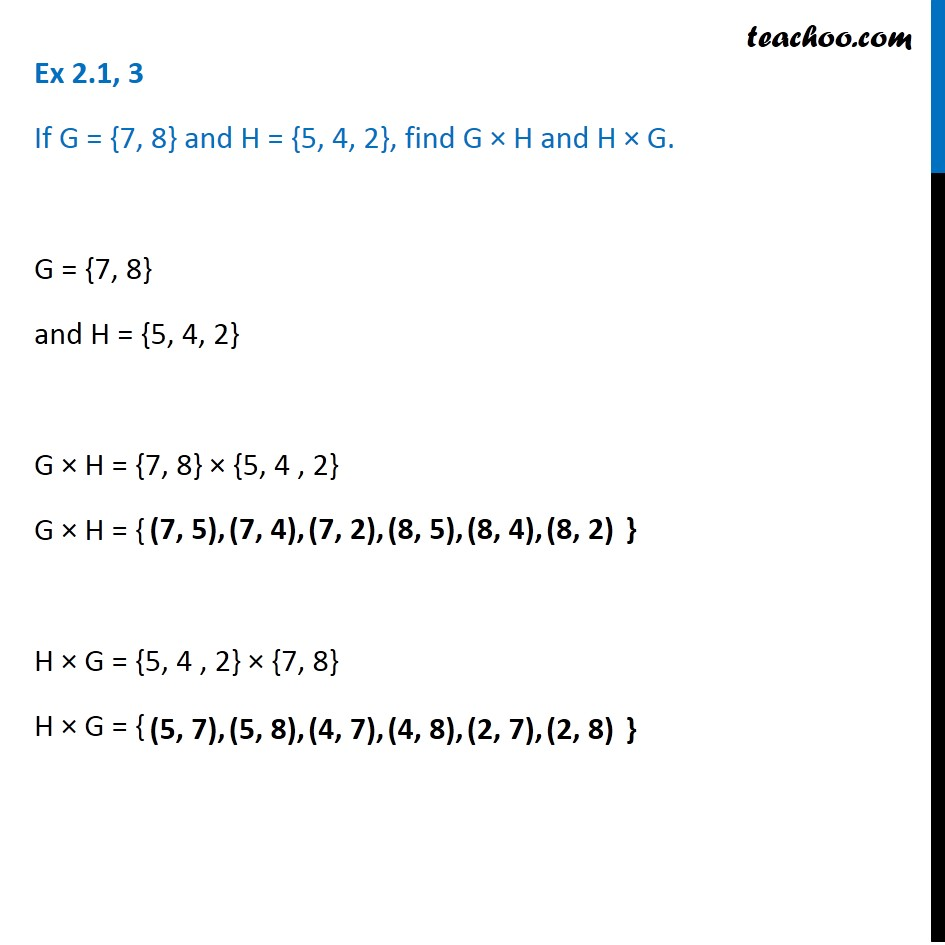Learn All Concepts of Chapter 2 Class 11 Relations and Function - FREE. Check - Relation and Function Class 11 - All Concepts1. Chapter 2 Class 11 Relations and Functions
2. Serial order wise
3. Ex 2.1

Transcript

Ex 2.1, 3 If G = {7, 8} and H = {5, 4, 2}, find G × H and H × G. G = {7, 8} and H = {5, 4, 2} G × H = {7, 8} × {5, 4 , 2} G × H = { H × G = {5, 4 , 2} × {7, 8} H × G = { (7, 5), (5, 7), (5, 8), (4, 7), (2, 7), (2, 8)

Ex 2.1